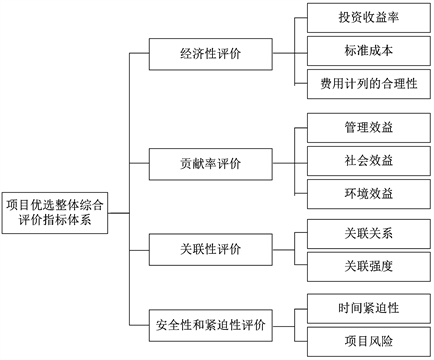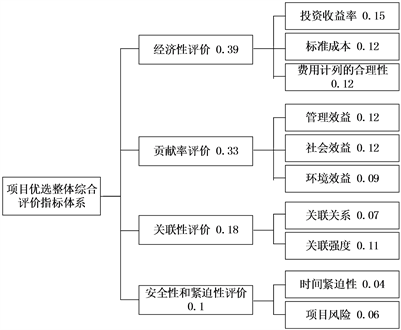储备项目关联集群的优先级评价模型研究Research on Priority Evaluation Model of Relevant Cluster of Reserve Projects

• 全文下载: PDF(532KB)    PP.40-50   DOI: 10.12677/MM.2019.91005
• 下载量: 121  浏览量: 226

With the deepening of the reform of the electric power system, the demand for accurate investment of power grid enterprises is getting higher and higher. Faced with the pressure of the situation, how to improve efficiency and efficiency, reduce the scale of investment, improve the accuracy of investment arrangements and implement accurate management from the source has become the focus of attention of power grid enterprises. In this paper, through the cluster demonstration of the integrated planning project, we can strengthen the management and control of the reserve project in advance from the source, and improve the quality and efficiency of the integrated planning management. Firstly, this paper classifies projects and establishes related clusters from the perspective of business relevance. Secondly, on the basis of the establishment of project related clusters, it puts forward demonstration methods and measures from three aspects: normativeness, synergy, efficiency and benefit. Finally, it builds a priority evaluation model of project related clusters with the help of portfolio management theory. From the strategic and policy point of view, the project portfolio should be selected to maximize the benefits of the project.

1. 引言

2. 综合计划储备项目的关联类型分析

1) 购置建设关联。购置建设关联是指建设、改造等工程项目与未包含在工程内的配套设施设备购置项目相互关联，目的在于方便开展投资统计，合理安排项目进度，协调项目施工。

2) 建设资产关联。建设资产关联是指在同一地点施工或对同一设施设备施工的项目相互关联，旨在筛查重复投资，或查找因规避限上项目审批而进行的储备项目拆分行为。

3) 服务群体关联。服务群体关联是指在输配电环节以外为同一客户、客户群或同一类员工提供服务的项目，目的在于统一投资思路，删除重复投资或不合理投资，进行统一调配和管控，协调项目进度。3. 项目关联集群论证

1) 规范性论证

2) 协同性论证

3) 效率效益论证

4) 重复性筛查

4. 项目优先级评价模型的构建

4.1. 项目组合管理理论Figure 1. Evaluation index system of project optimum selection

4.2. 评价体系的建立

4.3. 项目组合的优选模型

1) 根据指标体系，确定评价标准

2) 确定评价指标 ${U}_{i}$${V}_{ij}$ 的权重

3) 组织评价专家评分

4) 求评价样本矩阵

$\begin{array}{l}\text{\hspace{0.17em}}\text{\hspace{0.17em}}\text{\hspace{0.17em}}\text{\hspace{0.17em}}\text{\hspace{0.17em}}\text{\hspace{0.17em}}\text{\hspace{0.17em}}\text{\hspace{0.17em}}\text{\hspace{0.17em}}\text{\hspace{0.17em}}\text{\hspace{0.17em}}\text{\hspace{0.17em}}\text{\hspace{0.17em}}1\text{\hspace{0.17em}}\text{\hspace{0.17em}}\text{\hspace{0.17em}}\text{\hspace{0.17em}}\text{\hspace{0.17em}}\text{\hspace{0.17em}}\text{\hspace{0.17em}}\text{\hspace{0.17em}}\text{\hspace{0.17em}}2\text{\hspace{0.17em}}\text{\hspace{0.17em}}\text{\hspace{0.17em}}\text{\hspace{0.17em}}\text{\hspace{0.17em}}\text{\hspace{0.17em}}\cdots \text{\hspace{0.17em}}\text{\hspace{0.17em}}\text{\hspace{0.17em}}\text{\hspace{0.17em}}\text{\hspace{0.17em}}\text{\hspace{0.17em}}p\\ {D}^{\left(S\right)}=\left[\begin{array}{ccccc}{d}_{111}^{\left(S\right)}& {d}_{111}^{\left(S\right)}& \cdots & {d}_{111}^{\left(S\right)}& {V}_{11}\\ {d}_{121}^{\left(S\right)}& {d}_{122}^{\left(S\right)}& \cdots & {d}_{12p}^{\left(S\right)}& {V}_{12}\\ ⋮& ⋮& \ddots & ⋮& ⋮\\ {d}_{1{n}_{1}1}^{\left(S\right)}& {d}_{1{n}_{1}2}^{\left(S\right)}& \cdots & {d}_{1{n}_{1}p}^{\left(S\right)}& {V}_{1{n}_{1}}\\ {d}_{211}^{\left(S\right)}& {d}_{212}^{\left(S\right)}& \cdots & {d}_{21p}^{\left(S\right)}& {V}_{21}\\ {d}_{221}^{\left(S\right)}& {d}_{222}^{\left(S\right)}& \cdots & {d}_{22p}^{\left(S\right)}& {V}_{22}\\ ⋮& ⋮& \ddots & ⋮& ⋮\\ {d}_{2{n}_{2}1}^{\left(S\right)}& {d}_{2{n}_{2}2}^{\left(S\right)}& \cdots & {d}_{2{n}_{2}p}^{\left(S\right)}& {V}_{2{n}_{1}}\\ ⋮& ⋮& \ddots & ⋮& ⋮\\ {d}_{m11}^{\left(S\right)}& {d}_{m12}^{\left(S\right)}& \cdots & {d}_{m1p}^{\left(S\right)}& {V}_{m1}\\ {d}_{m21}^{\left(S\right)}& {d}_{m22}^{\left(S\right)}& \cdots & {d}_{m2p}^{\left(S\right)}& {V}_{m2}\\ ⋮& ⋮& \ddots & ⋮& ⋮\\ {d}_{m{n}_{m}1}^{\left(S\right)}& {d}_{m{n}_{m}2}^{\left(S\right)}& \cdots & {d}_{m{n}_{m}p}^{\left(S\right)}& {V}_{m{n}_{m}}\end{array}\right]=\left({d}_{ijk}^{\left(S\right)}\right)p\sum {n}_{i}\end{array}$

$i=1,2,\cdots ,m;j=1,2,\cdots ,{n}_{m};k=1,2,\cdots ,p$

5) 确定评价灰类

$e=1$ 时，灰类为“优”，即 ${\otimes }_{1}\in \left(4,\infty \right)$，其表达式为：

${f}_{1}\left({d}_{ijk}^{\left(S\right)}\right)=\left\{\begin{array}{l}\frac{{d}_{ijk}^{\left(S\right)}}{4},\text{\hspace{0.17em}}\text{\hspace{0.17em}}\text{\hspace{0.17em}}{d}_{ijk}^{\left(S\right)}\in \left(0,4\right)\\ 1,\text{\hspace{0.17em}}\text{\hspace{0.17em}}\text{\hspace{0.17em}}\text{\hspace{0.17em}}\text{\hspace{0.17em}}\text{\hspace{0.17em}}\text{\hspace{0.17em}}\text{\hspace{0.17em}}{d}_{ijk}^{\left(S\right)}\in \left(4,\infty \right)\\ 0,\text{\hspace{0.17em}}\text{\hspace{0.17em}}\text{\hspace{0.17em}}\text{\hspace{0.17em}}\text{\hspace{0.17em}}\text{\hspace{0.17em}}\text{\hspace{0.17em}}\text{ }{d}_{ijk}^{\left(S\right)}\stackrel{¯}{\in }\left(0,\infty \right)\end{array}$

$e=2$ 时，灰类为“良”，即 ${\otimes }_{2}\in \left(0,3,6\right)$，其表达式为：

${f}_{2}\left({d}_{ijk}^{\left(S\right)}\right)=\left\{\begin{array}{l}\frac{{d}_{ijk}^{\left(S\right)}}{3},\text{\hspace{0.17em}}\text{\hspace{0.17em}}\text{\hspace{0.17em}}\text{\hspace{0.17em}}\text{\hspace{0.17em}}\text{\hspace{0.17em}}\text{\hspace{0.17em}}\text{\hspace{0.17em}}{d}_{ijk}^{\left(S\right)}\in \left(0,3\right)\\ \frac{{d}_{ijk}^{\left(S\right)}-6}{-3},\text{\hspace{0.17em}}\text{\hspace{0.17em}}\text{\hspace{0.17em}}{d}_{ijk}^{\left(S\right)}\in \left(3,6\right)\\ 0,\text{\hspace{0.17em}}\text{\hspace{0.17em}}\text{\hspace{0.17em}}\text{\hspace{0.17em}}\text{\hspace{0.17em}}\text{\hspace{0.17em}}\text{\hspace{0.17em}}\text{\hspace{0.17em}}\text{ }\text{\hspace{0.17em}}\text{\hspace{0.17em}}\text{\hspace{0.17em}}\text{\hspace{0.17em}}{d}_{ijk}^{\left(S\right)}\stackrel{¯}{\in }\left(0,6\right)\end{array}$

$e=3$ 时，灰类为“中”，即 ${\otimes }_{3}\in \left(0,2,4\right)$，其表达式为：

${f}_{3}\left({d}_{ijk}^{\left(S\right)}\right)=\left\{\begin{array}{l}\frac{{d}_{ijk}^{\left(S\right)}}{2},\text{\hspace{0.17em}}\text{\hspace{0.17em}}\text{\hspace{0.17em}}\text{\hspace{0.17em}}\text{\hspace{0.17em}}\text{\hspace{0.17em}}\text{\hspace{0.17em}}\text{\hspace{0.17em}}{d}_{ijk}^{\left(S\right)}\in \left(0,2\right)\\ \frac{{d}_{ijk}^{\left(S\right)}-4}{-2},\text{\hspace{0.17em}}\text{\hspace{0.17em}}\text{\hspace{0.17em}}{d}_{ijk}^{\left(S\right)}\in \left(2,4\right)\\ 0,\text{\hspace{0.17em}}\text{\hspace{0.17em}}\text{\hspace{0.17em}}\text{\hspace{0.17em}}\text{\hspace{0.17em}}\text{\hspace{0.17em}}\text{\hspace{0.17em}}\text{\hspace{0.17em}}\text{\hspace{0.17em}}\text{\hspace{0.17em}}\text{ }\text{\hspace{0.17em}}\text{\hspace{0.17em}}{d}_{ijk}^{\left(S\right)}\stackrel{¯}{\in }\left(0,4\right)\end{array}$

$e=4$ 时，灰类为“差”，即 ${\otimes }_{4}\in \left(0,1,2\right)$，其表达式为：

${f}_{4}\left({d}_{ijk}^{\left(S\right)}\right)=\left\{\begin{array}{l}1,\text{\hspace{0.17em}}\text{\hspace{0.17em}}\text{\hspace{0.17em}}\text{\hspace{0.17em}}\text{\hspace{0.17em}}\text{\hspace{0.17em}}\text{\hspace{0.17em}}\text{\hspace{0.17em}}\text{\hspace{0.17em}}\text{\hspace{0.17em}}\text{\hspace{0.17em}}\text{\hspace{0.17em}}\text{\hspace{0.17em}}{d}_{ijk}^{\left(S\right)}\in \left(0,1\right)\\ \frac{{d}_{ijk}^{\left(S\right)}-2}{-1},\text{\hspace{0.17em}}\text{\hspace{0.17em}}\text{\hspace{0.17em}}{d}_{ijk}^{\left(S\right)}\in \left(1,2\right)\\ 0,\text{\hspace{0.17em}}\text{\hspace{0.17em}}\text{\hspace{0.17em}}\text{\hspace{0.17em}}\text{\hspace{0.17em}}\text{\hspace{0.17em}}\text{\hspace{0.17em}}\text{\hspace{0.17em}}\text{\hspace{0.17em}}\text{\hspace{0.17em}}\text{\hspace{0.17em}}\text{\hspace{0.17em}}{d}_{ijk}^{\left(S\right)}\stackrel{¯}{\in }\left(0,2\right)\end{array}$

6) 计算灰色评价系数 

7) 计算灰色评价权向量及权矩阵 

8) 对 ${V}_{i}$ 作综合评价

9) 对U作综合评价

${V}_{i}$ 的综合评价结果 ${B}_{i}^{\left(S\right)}$ 得第S个项目的U所属指标 ${U}_{i}$。对于灰色评价权矩阵 ${R}^{\left(S\right)}=\left({B}_{1}^{\left(S\right)},{B}_{2}^{\left(S\right)},\cdots ,{B}_{m}^{\left(S\right)}\right)$，则第S个项目的指标U的综合评价结果为 ${B}^{\left(S\right)}=A{R}^{\left(S\right)}$

10) 计算综合评价值

$e=1$ 时取4， $e=2$ 时取3， $e=3$ 时取2， $e=4$ 时取1，则各评价灰类等级值化向量 $C=\left(4,3,2,1\right)$${W}^{\left(S\right)}={B}^{\left(S\right)}{C}^{\text{T}}$ 为对第S个项目进行综合评价的评价值  。

11) 确定项目集群优先级

5. 模型分析与讨论

1) 确定二级评价指标 ${V}_{ij}$ 的评分等级标准

2) 运用层次分析法确定一级评价指标 ${U}_{i}$ 和二级评价指标 ${V}_{ij}$ 的权重Table 3. Importance comparison scaleFigure 2. Weights of two-level indicators

3) 组织评价专家评分

4) 求评价样本矩阵

${D}^{\left(1\right)}=\left(\begin{array}{cccccc}2.5& 3& 2& 2.5& 4& 3\\ 3& 3& 3& 3& 3.5& 3\\ 2& 3& 3& 3& 3& 2.5\\ 3& 3.5& 2.5& 3.5& 2& 3.5\\ 2.5& 2.5& 2& 3& 3& 2.5\\ 3& 2.5& 3& 3& 3& 3\\ 2.5& 3.5& 3& 3& 3& 2\\ 2.5& 3& 3& 3& 3& 2.5\\ 3& 3& 2.5& 3& 3& 3\\ 3.5& 3& 2& 2.5& 2.5& 3\end{array}\right)$

5) 确定评价灰类

6) 计算灰色评价系数

$e=1$

$\begin{array}{c}{x}_{111}^{\left(1\right)}={\sum }_{k=1}^{6}{f}_{1}\left({d}_{11k}^{\left(1\right)}\right)={f}_{1}\left(2.5\right)+{f}_{1}\left(3\right)+{f}_{1}\left(2\right)+{f}_{1}\left(2.5\right)+{f}_{1}\left(4\right)+{f}_{1}\left(3\right)\\ =0.625+0.75+0.5+0.625+1+0.75=4.25\end{array}$

$e=2$

$\begin{array}{c}{x}_{112}^{\left(1\right)}={\sum }_{k=1}^{6}{f}_{2}\left({d}_{11k}^{\left(1\right)}\right)={f}_{2}\left(2.5\right)+{f}_{2}\left(3\right)+{f}_{2}\left(2\right)+{f}_{2}\left(2.5\right)+{f}_{2}\left(4\right)+{f}_{2}\left(3\right)\\ =0.8333+1+0.6667+0.8333+0.6667+1=5\end{array}$

$e=3$

$\begin{array}{c}{x}_{113}^{\left(1\right)}={\sum }_{k=1}^{6}{f}_{3}\left({d}_{11k}^{\left(1\right)}\right)={f}_{3}\left(2.5\right)+{f}_{3}\left(3\right)+{f}_{3}\left(2\right)+{f}_{3}\left(2.5\right)+{f}_{3}\left(4\right)+{f}_{3}\left(3\right)\\ =0.75+0.5+1+0.75+0+0.5=3.5\end{array}$

$e=4$

${x}_{114}^{\left(1\right)}={\sum }_{k=1}^{6}{f}_{4}\left({d}_{11k}^{\left(1\right)}\right)={f}_{4}\left(2.5\right)+{f}_{4}\left(3\right)+{f}_{4}\left(2\right)+{f}_{4}\left(2.5\right)+{f}_{4}\left(4\right)+{f}_{4}\left(3\right)=0$

${x}_{11}^{\left(1\right)}=\underset{e=1}{\overset{4}{\sum }}{x}_{11e}^{\left(1\right)}={x}_{112}^{\left(1\right)}+{x}_{112}^{\left(1\right)}+{x}_{112}^{\left(1\right)}+{x}_{112}^{\left(1\right)}=12.75$

7) 计算灰色评价权向量及权矩阵

$e=1$ ${r}_{111}^{\left(1\right)}=\frac{{x}_{111}^{\left(1\right)}}{{x}_{11}^{\left(1\right)}}=0.333$

$e=2$ ${r}_{112}^{\left(1\right)}=\frac{{x}_{112}^{\left(1\right)}}{{x}_{11}^{\left(1\right)}}=0.408$

$e=3$ ${r}_{113}^{\left(1\right)}=\frac{{x}_{113}^{\left(1\right)}}{{x}_{11}^{\left(1\right)}}=0.259$

$e=4$ ${r}_{114}^{\left(1\right)}=\frac{{x}_{114}^{\left(1\right)}}{{x}_{11}^{\left(1\right)}}=0$

${r}_{11}^{\left(1\right)}=\left({r}_{111}^{\left(1\right)},{r}_{112}^{\left(1\right)},{r}_{113}^{\left(1\right)},{r}_{114}^{\left(1\right)}\right)=\left(0.333,0.408,0.259,0\right)$

${R}_{1}^{\left(1\right)}=\left(\begin{array}{c}{r}_{11}^{\left(1\right)}\\ {r}_{12}^{\left(1\right)}\end{array}\right)=\left(\begin{array}{cccc}0.333& 0.408& 0.259& 0\\ 0.35& 0.442& 0.208& 0\end{array}\right)$

${R}_{2}^{\left(1\right)}=\left(\begin{array}{c}{r}_{21}^{\left(1\right)}\\ {r}_{22}^{\left(1\right)}\\ {r}_{23}^{\left(1\right)}\end{array}\right)=\left(\begin{array}{cccc}0.325& 0.408& 0.267& 0\\ 0.317& 0.422& 0.261& 0\\ 0.325& 0.433& 0.242& 0\end{array}\right)$

${R}_{3}^{\left(1\right)}=\left(\begin{array}{c}{r}_{31}^{\left(1\right)}\\ {r}_{32}^{\left(1\right)}\end{array}\right)=\left(\begin{array}{cccc}0.307& 0.356& 0.337& 0\\ 0.301& 0.429& 0.27& 0\end{array}\right)$

${R}_{4}^{\left(1\right)}=\left(\begin{array}{c}{r}_{41}^{\left(1\right)}\\ {r}_{42}^{\left(1\right)}\end{array}\right)=\left(\begin{array}{cccc}0.389& 0.379& 0.232& 0\\ 0.283& 0.377& 0.34& 0\end{array}\right)$

8) 对一级指标 ${U}_{i}$ 进行综合评价

${B}_{1}^{\left(1\right)}={A}_{1}\cdot {R}_{1}^{\left(1\right)}=\left(0.3254,0.4114,0.2632,0\right)$

${B}_{2}^{\left(1\right)}={A}_{1}\cdot {R}_{1}^{\left(1\right)}=\left(0.3374,0.4074,0.2552,0\right)$

${B}_{3}^{\left(1\right)}={A}_{1}\cdot {R}_{1}^{\left(1\right)}=\left(0.\text{3261},0.\text{3782},0.\text{2957},0\right)$

${B}_{4}^{\left(1\right)}={A}_{1}\cdot {R}_{1}^{\left(1\right)}=\left(0.\text{3466},0.\text{3782},0.\text{2752},0\right)$

9) 对项目优先级进行综合评价

${B}_{1}^{\left(1\right)}$${B}_{2}^{\left(1\right)}$${B}_{3}^{\left(1\right)}$${B}_{4}^{\left(1\right)}$ 得项目1的总灰色评价权矩阵 ${R}^{\left(1\right)}$

${R}^{\left(1\right)}=\left(\begin{array}{c}{B}_{1}^{\left(1\right)}\\ {B}_{2}^{\left(1\right)}\\ {B}_{3}^{\left(1\right)}\\ {B}_{4}^{\left(1\right)}\end{array}\right)=\left(\begin{array}{cccc}0.3254& 0.4114& 0.2632& 0\\ 0.3374& 0.4074& 0.2552& 0\\ 0.3261& 0.3782& 0.2957& 0\\ 0.3466& 0.3782& 0.2752& 0\end{array}\right)$

10) 计算综合评价值并排序

${W}^{\left(1\right)}={B}^{\left(1\right)}\cdot {C}^{\text{T}}=\left(0.3316,0.4008,0.2676,0\right)\cdot {\left(4,3,2,1\right)}^{\text{T}}=3.064$

${W}^{\left(2\right)}=3.315;\text{\hspace{0.17em}}\text{\hspace{0.17em}}{W}^{\left(3\right)}=3.137$

11) 确定项目集群优先级

6. 小结

  曾玉成, 王俊川, 任佩瑜. 基于企业战略的项目组合管理流程研究[J]. 统计与决策, 2010(9): 177-180.  乔卫国. 电网工程投资评价与组合优化研究[D]: [硕士学位论文]. 北京: 华北电力大学, 2015.  许菲. 企业战略导向、项目组合管理与项目组合成功的关系研究[D]: [硕士学位论文]. 天津: 南开大学, 2012.  胡海华. 社会网络强弱关系对农业技术扩散的影响——从个体到系统的视角[J]. 华中农业大学学报(社会科学版), 2016(5): 47-54.  张浩. 城市轨道交通项目关系网络风险研究[D]: [硕士学位论文]. 石家庄: 石家庄铁道大学, 2017.  许文秀. 电网建设项目经济评价模型及储备库优化研究[D]: [硕士学位论文]. 北京: 华北电力大学, 2012.  张丽. 企业级项目组合优选研究[D]: [硕士学位论文]. 长沙: 湖南大学, 2007.  吴燕. 基于层次聚类的科技项目分类与查重研究[D]: [硕士学位论文]. 天津: 天津财经大学, 2008.  徐永其, 胡志键. 战略导向的项目评价指标体系的构建[Z]. 青岛: 中国技术经济论坛, 2008.  肖依永, 常文兵, 郭伟宏. 基于关联规则的ABC库存分类方法[J]. 系统工程, 2008, 26(6): 10-15.